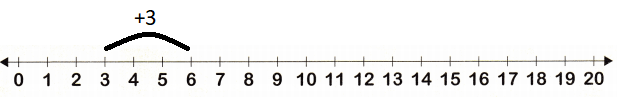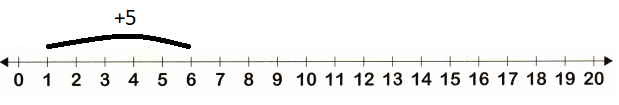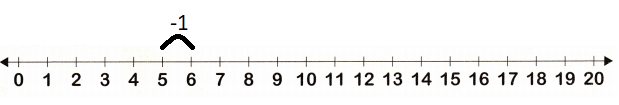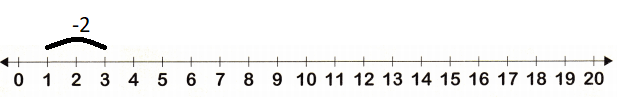# McGraw Hill Math Grade 1 Chapters 1–6 Review Test Answer Key

All the solutions provided in McGraw Hill Math Grade 1 Answer Key PDF Chapter 1-6 Review Test as per the latest syllabus guidelines.

Tell how many. Write the number.

Question 1.________
3
Explanation:
There are 3 frogs in all.

Question 2.________
5
Explanation:
There are 5 pigs in all.

Question 3.________
7
Explanation:
there are 7 horses in all.

Question 4.________
15
Explanation:
There are 20 squares
15 blue dots in 20 squares.

Question 5.________
20 yellow dots
Explanation:
There are 20 squares
20 yellow dots in 20 squares.

Question 6.________
6
Explanation:
There are 6 fishes in all.

Question 7.________
4
Explanation:
There are 4 pink birds

Question 8.________
2
Explanation:
There are 2 honey bees.

Question 9.________
16 cubes
Explanation:
5 cubes of 3 sticks and 1 cube
5 x 3 = 15
15 + 1 = 16.

Question 10.________
6
Explanation:
1 stick of 5 cubes and 1 cube
5 + 1 = 6

Add to find out how many. Write the sum. Use the number line to help.Question 11.3 + 2 = ___ oranges
5Explanation:
3 + 2 = 5 oranges
The sum of 3 and 2 oranges = 5 oranges.

Question 12.3 + 3 = _____ lemons3 + 3 = 6 lemons
Explanation:
The sum of 3 and 3 is 6 lemons

Question 13.1 + 5 = ___ apples1 + 5 = 6 apples
Explanation:
The sum of 1 and 5 is 6 apples.

Question 14.2 + 0 = ___ cherries
2 + 0 = 2 cherries
Explanation:
the sum of 2 and 0 is 2 cherries.

Question 15.
2 + 1 = ___
3
Explanation:
2 + 1 = 3
The sum of 2 and 1 is 3

Question 16.
5 + 3 = ___
8
Explanation:
5 + 3 = 8
The sum of 5 and 3 is 8

Question 17.
6 + 7 = ___
13
Explanation:
6 + 7 = 13
The sum of 6 and 7 is 13

Question 18.
4 + 6 = ___
10
Explanation:
4 + 6 = 10
The sum of 4 and 6 is 10.

Question 19.
9 + 2 = ___
11
Explanation:
9 + 2 = 11
The sum of 9 and 2 is 11.

Question 20.
8 + 4 = ___
12
Explanation:
8 + 4 = 12
The sum of 8 and 4 is 12.

Question 21.
7 + 13 = ___
20
Explanation:
7 + 13 = 20
The sum of 7 and 13 is 20.

Question 22.
1 + 19 = ___
20
Explanation:
1 + 19 = 20

Subtract. Write the difference. Use the number line to help.Question 23.6 – 1 = ___
5Explanation:
The difference of 6 and 1 is 5
6 – 1 = 5

Question 24.3 – 2 = ___
1
Explanation:The difference of 3 and 2 is 1
3 – 2 = 1

Question 25.
4 – 3 = ___
1Explanation:
The difference of 4 and 3 is 1
4 – 3 = 1

Question 26.
13 – 0 = ___
13
Explanation:
The difference of 13 and 0 is 13
13 – 0 = 13

Question 27.
12 – 5 = ___
7
Explanation:
The difference of 12 and 5 is 7
12 – 5 = 7

Question 28.
8 – 8 = ___
0
Explanation:
8 – 8 = 0
The difference of 8 and 8 is 0

Question 29.5 – 3 = ___
2
Explanation:
5 – 3 = 2
The difference of 5 and 3 is 2

Question 30.4 – 3 = ___
1
Explanation:
4 – 3 = 1
The difference of 4 and 3 is 1

Question 31.
19 – 11 = ___
8
Explanation:
19 – 11 = 8
The difference of 19 and 11 is 8.

Question 32.
15 – 8 = ___
7
Explanation:
15 – 8 = 7
The difference of 15 and 8 is 7

Question 33.
6 – 4 = ___
2
Explanation:
6 – 4 = 2
The difference of 6 and 4 is 2.

Question 34.
17 – 0 = ___
17
Explanation:
The difference of 17 and 0 is 17.
17 – 0 = 17

Count by 1s, 5s, or 10s. Write the missing numbers.

Question 35.
5 6 7 ___ ___ ___ ___ 12
5 6 7 8 9 10 11 12
Explanation:
The given series is skip by ones
we have to add one to the given number
7 + 1 = 8
8 + 1 = 9

Question 36.
65 70 ___ ___ 85 ___ _____
65 70 75 80 85 90 95
Explanation:
The given series is skip by fives.
70 + 5 = 75
75 + 5 = 80

Question 37.
10 20 _____ 40 _____ _____ _____
10 20 30 40 50 60 70
Explanation:
The given series is skip by tens
20 + 10 = 30
40 + 10 = 50

Question 38.
25 30 ___ ___ ___ ____ 55
25 30 35 40 45 50 55
Explanation:
The given series is skip by fives
30 + 5 = 35
35 + 5 = 40

Solve. Write the sum or difference.

Question 39.
Elsa has 4. Nate has 5. How many fish in all?
4 + 5 = _____ fish
9
Explanation:
Number of fishes that Elsa has 4.
Number of fishes that Nate has 5.
4 + 5 = 9 fish in all.

Question 40.
There are 8. 2leave. How many cars are left?
8 — 2 = _____ cars
6 cars
Explanation:
Number of cars are 8.
2leave.
8 — 2 = 6 cars are left

Question 41.
There are 19. 3fly away. How many birds are left?
19 – 3 = _____ birds
16 birds.
Explanation:
Number of  are 19.
3fly away.
19 – 3 = 16 birds are left

Question 42.
There are 7 red blocks and 7 green blocks. How many blocks in all?
7 + 7 = _____ blocks